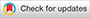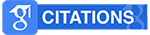# Pengaruh Model Pembelajaran Word Square terhadap Hasil Belajar IPS Siswa Kelas IV SDN Cipondoh 2 Kota Tangerang## Abstract

This study aims to determine the effect of the word square learning model on student learning outcomes in social studies subjects. This research was conducted at SDN Cipondoh 2 Tangerang City. This type of research is quantitative descriptive in the form of a quasi-experimental. The design used is Noneequivalent Control Group Design. Data collection techniques using SPSS and excel calculations. The samples in this study were students of class IV B as the experimental class totaling 30 students, and class IV A as the control class totaling 30 students. Based on the results of the study, the experimental class pretest results obtained a mean of 62.13, mode of 57.32 and median of 60.5. In the control class pretest results obtained mean 56.9, mode 57.83 and median 57.25. Then from the posttest results of the experimental class, the mean value was 78.9, the mode was 81 and the median was 80.17. In the posttest control class the mean is 72.5, the mode is 72.58 and the median is 73. Based on the hypothesis test, the results of the experimental class posttest are 2.743. Because the results of the hypothesis show 2.743 > 2.002 then Ho is accepted. This means that the word square model has an influence on the learning outcomes of fourth grade students at SDN Cipondoh 2 Tangerang City. And with the Word Square model, students study more carefully, learning becomes fun, not boring, very effectively applied at SDN Cipondoh 2 Tangerang City.

Keywords:
Learning Outcomes; Word Square Learning Model; Social Sciences

Citation Metrics: